﻿ Hybrid Block Method Algorithms for Solution of First Order Initial Value Problems in Ordinary Differential Equations

# Hybrid Block Method Algorithms for Solution of First Order Initial Value Problems in Ordinary Differential Equations

Ajileye G*, Amoo SA and Ogwumu OD
Department of Mathematics and Statistics, Federal University Wukari, Wukari, Taraba State, Nigeria
*Corresponding Author: Ajileye G, Department of Mathematics and Statistics, Federal University Wukari, Wukari, Taraba State, Nigeria, Tel: 2348034906427, Email: [email protected]

Received Date: Apr 16, 2018 / Accepted Date: May 30, 2018 / Published Date: Jun 19, 2018

Keywords: Collocation; Interpolation; Linear multistep method; Hybrid and power series polynomial

#### Introduction

In recent times, the integration of Ordinary Differential Equations (ODEs) is carried out using some kinds of block methods. In this paper, we propose an order six block integrator for the solution of first-order ODEs of the form: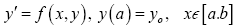(1)

Where f is continuous within the interval of integration [a,b]. We assume that f satisfies Lipchitz condition which guarantees the existence and uniqueness of solution of eqn. (1). For the discrete solution of (1) by linear multi-step method has being studied by authors like  and continuous solution of eqn. (1) and [2-4]. One important advantage of the continuous over discrete approach is the ability to provide discrete schemes for simultaneous integration. These discrete schemes can be reformulated as general linear methods (GLM) . The block methods are self-starting and can be applied to both stiff and non-stiff initial value problem in differential equations. More recently, authors like [6-10] and to mention few, these authors proposed methods ranging from predictor- corrector to hybrid block method for initial value problem in ordinary differential equation.

In this work, hybrid blocks method with two off-grid using Power series expansion [11,12]. This would help in coming up with a more computationally reliable integrator that could solve first order differential equations problems of the form eqn. (1).

#### Derivation of Hybrid Method

In this section, we intend to construct the proposed two-step LMMs which will be used to generate the method. We consider the power series polynomial of the form: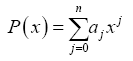(2)

which is used as our basis to produce an approximate solution to (1.0) as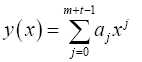(3)

And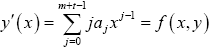(4)

where aj are the parameters to be determined, m and t are the points of collocation and interpolation respectively. This process leads to (m+t-1) of non-linear system of equations with (m+t-1) unknown coefficients, which are to be determined by the use of Maple 17 Mathematical software.

#### Hybrid Block Method

Using eqns. (3) and (4), m=1 and t=5 our choice of degree of polynomial is (m+t-1). Eqn. (3) is interpolates at the point x=xn and eqn. (4) is collocated at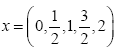which lead to system of equation of the form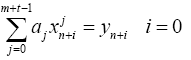(5)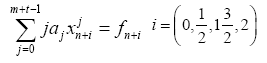(6)

With the mathematical software, we obtain the continuous formulation of eqns. (5) and (6) of the form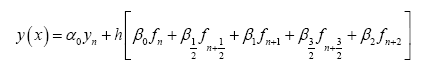(7)

After obtaining the values of αj and βj,j=0 and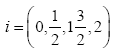in eqn. (7)

We evaluated at the point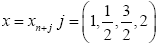which gives the following set of discrete schemes to form our hybrid block method.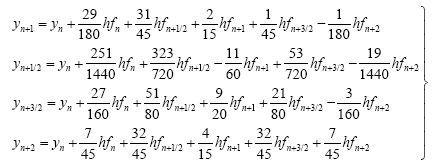(8)

Eqn. (8.0) are of uniform order 5, with error constant as follows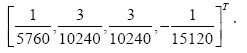Consistency

Definition: The Linear Multistep method is said to be consistent if it is of order

P≥ 1 and its first and second characteristic polynomial defined as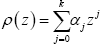and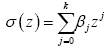where Z satisfies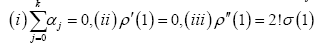See Lambart (1973).

The discrete Schemes derived are all of order than one and satisfy the condition (i)-(iii).

#### Zero Stability of the block Method

The block method is defined by Fatunla (1988) as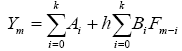where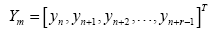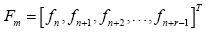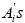and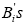are chosen r x r matrix coefficient and m=0,1,2… represents the block number, n=mγ the first step number in the m-th block and r is the proposed block size.

The block method is said to be zero stable if the roots of Rj,j=1(1)k of the first characteristics polynomial is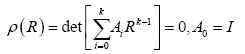satisfies |Rj|≤ 1, if one of the roots is +1, then the root is called Principal Root of ρ(R).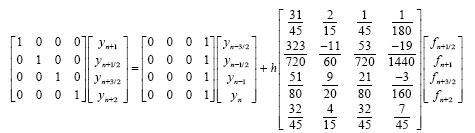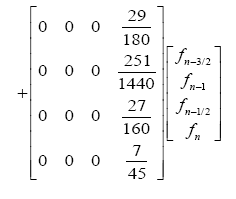Where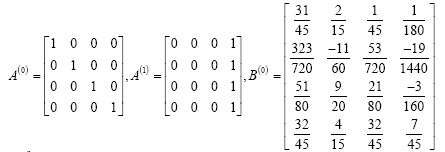and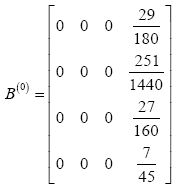The first characteristics polynomial of the scheme is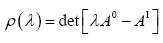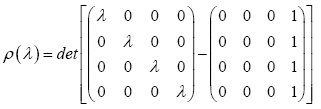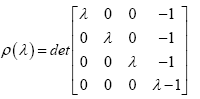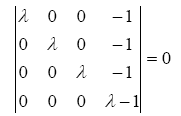λ3(λ-1)=0

λ123=0 or λ4=1

We can see clearly that no root has modulus greater than one (i.e λi≤1))∀i . The hybrid block method is zero stable.

#### Numerical Examples

Problem 1

y'= y, y (0) =1, h = 0.1

Exact solution

y(x)=exp(x) (Table 1).

x Exact Solution  Scheme  Error in Scheme Error 
0.1 1.105170918075648 1.105170917860730 2.149179E-10 1.226221039551945e-05
0.2 1.221402758160170 1.221402757685120 4.7505E-10 1.355183832019158e-05
0.3 1.349858807576003 1.349858806788490 7.875129E-10 1.497709759790133e-05
0.4 1.491824697641270 1.491824696480820 1.16045E-09 1.655225270247307e-05
0.5 1.648721270700128 1.648721269097010 1.603118E-09 1.829306831546695e-05
0.6 1.822118800390509 1.822118798264440 2.126069E-09 2.021696710463594e-05
0.7 2.013752707470477 2.013752704729200 2.741277E-09 2.234320409577606e-05
0.8 2.225540928492468 2.225540925030090 5.989459E-09 2.469305938346267e-05
0.9 2.459603111156950 2.459603106852120 4.30483-09 2.729005110868599e-05
1.0 2.718281828459046 2.718281824122030 4.337016E-09 3.01601708376864e-05

Table 1: Comparison of approximate solution of problem 1.

Problem 2

y'= 0.5(1− y), y (0) = 0.5, h = 0.1

Exact solution

y(x)=1-0.5e-0.5x (Table 2).

x Exact Solution  Scheme  Error in Scheme Error 
0.1 0.524385287749643 0.524385287750861 1.218026E-13 5.574430e-012
0.2 0.547581290982020 0.547581290981880 1.399991E-13 3.946177e-012
0.3 0.569646011787471 0.569646011786286 1.184941E-12 8.183232e-012
0.4 0.590634623461009 0.590634623462548 1.538991E-12 3.436118e-011
0.5 0.610599608464297 0.610599608463187 1.110001E-12 1.929743e-010
0.6 0.629590889659141 0.629590889658614 5.270229E-12 1.879040e-010
0.7 0.647655955140643 0.647655955142752 2.10898E-12 1.776835e-010
0.8 0.664839976982180 0.664839976969201 1.297895E-11 1.724676e-010
0.9 0.681185924189113 0.681185924158290 3.08229E-11 1.847545e-010
1.0 0.696734670143683 0.696734670139561 4.121925E-11 3.005770e-010

Table 2: Comparison of approximate solution of problem 2.

#### Discussion of Result

We observed that from the two problems tested with this proposed block hybrid method the results converges to exact solutions and also compared favorably with the existing similar methods (see Tables 1 and 2).

#### Conclusion

In this paper, we have presented Hybrid block method algorithm for the solution of first order ordinary differential equations. The approximate solution adopted in this research produced a block method with stability region. This made it to perform well on problems. The block method proposed was found to be zero-stable, consistent and convergent.

#### References

Citation: Ajileye G, Amoo SA, Ogwumu OD (2018) Hybrid Block Method Algorithms for Solution of First Order Initial Value Problems in Ordinary Differential Equations. J Appl Computat Math 7: 390. DOI: 10.4172/2168-9679.1000390

Copyright: © 2018 Ajileye G, et al. This is an open-access article distributed under the terms of the Creative Commons Attribution License, which permits unrestricted use, distribution, and reproduction in any medium, provided the original author and source are credited.

Select your language of interest to view the total content in your interested language

##### Recommended Journals
Viewmore
###### Article Usage
• Total views: 1722
• [From(publication date): 0-2018 - Dec 15, 2019]
• Breakdown by view type
• HTML page views: 1527Can't read the image? click here to refresh